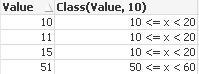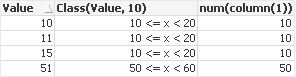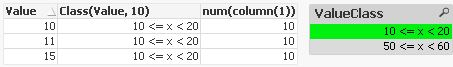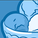# QlikView App Dev

Discussion Board for collaboration related to QlikView App Development.

Announcements
On May 18th at 10AM EDT we will answer your QlikView questions live. REGISTER
cancel
Showing results for
Did you mean:Employee

## How to use Class() function

A reoccurring question to Qlik Support has been how values can be grouped in intervals.

The simple answer to this question is to use the Class() function, which groups values into intervals.

For example the values below can be grouped in to intervals of 10, which means that the values 10, 11 and 15 all belong to the same interval of 10 to 20. The value 51 on the other hand fits in the group 50 to 60.There are no values between 20 and 50 so no other groups are generated.As all other values the classified interval label is a dual value, and has a underlying numerical value. This can be seen by the right alignment of the interval labels. By using the Num() function it is also possible to reveal the actual underlying value, and see that all intervals are numerically represented by the intervals first value.The same Class() based interval classification can be done in the load script, and thereby a pre-calculated interval has been generated. As any other field by selecting in the interval field in the front-end application, it is now possible to make an interval based selection.Specialist

class(expression, interval [ , label [ , offset ]])

Creates a classification of expressions. The bin width is determined by the number set as interval. The result is shown as a<=x<b, where a and b are the upper and lower limits of the bin. The x can be replaced by an arbitrary string stated in label. 0 is normally the default starting point of the classification. This can be changed by adding an offset.

Examples:

class( var,10 ) with var = 23 returns '20<=x<30'

class( var,5,'value' ) with var = 23 returns '20<= value <25'

class( var,10,'x',5 ) with var = 23 returns '15<=x<25'